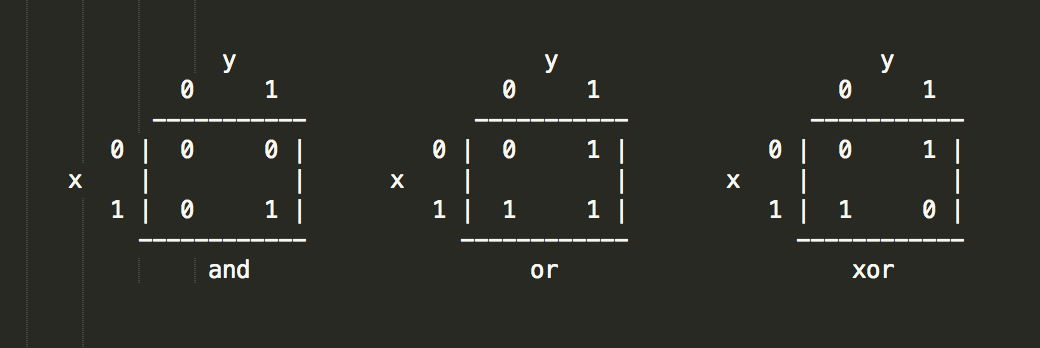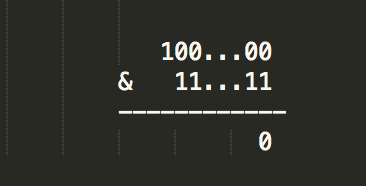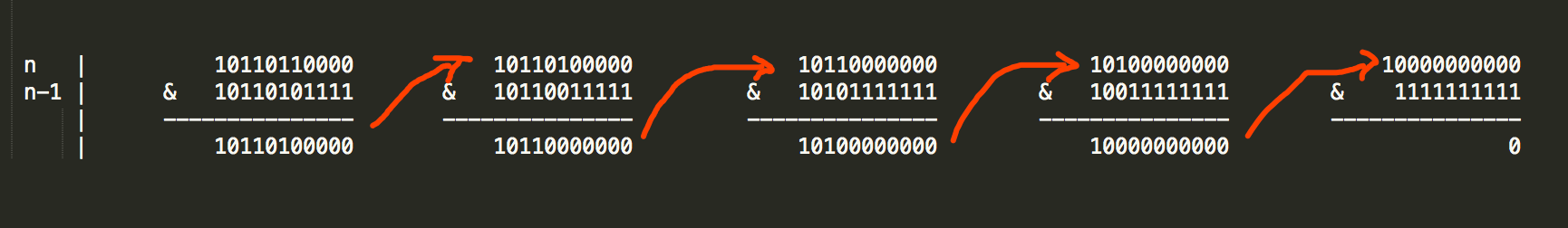# 位运算的应用技巧（图1）

## 1 判断奇偶数

``````bool IsOddNumber(unsigned int num)
{
return num & 1;
}``````

## 2 判断是否是 2 的幂

``````bool IsPowerOfTwo(unsigned int num)
{
return (num & (num-1)) == 0;
}``````（图2）

## 3 数字二进制表示，1 的数目

``````unsigned int GetOneCount(unsigned int num)
{
unsigned int count = 0;
while (num > 0) {
count += 1;
num = num & (num-1);
}

return count;
}``````(图3)

## 4 整数A转换为B，需要改变多少个二进制位

``````unsigned int GetDifferentBitCount(unsigned int a, unsigned int b)
{
unsigned int t = a ^ b;
return GetOneCount(t);
}``````

## 5 交换

``````#define SWAP(a, b) (((a) ^= (b)), ((b) ^= (a)), ((a) ^= (b)))
#define SWAP(a, b) (((a) == (b)) || (((a) ^= (b)), ((b) ^= (a)), ((a) ^= (b))))``````

## 6 一个数组内的数字，只有一个出现一次，其他的出现两次。找出出现一次的那个数字

``````int GetSingleNumber(int addr[], unsigned int size)
{
int t = 0;
unsigned int i = 0;
for (i = 0; i < size; i++) {